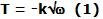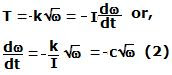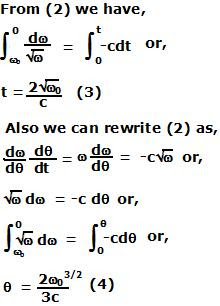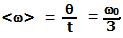Sunday, November 16, 2008

Irodov Problem 1.250

Since the torque (moment) due to all forces acting on the flywheel is proportional to the square root of its angular velocity, let this moment be,where k is some constant. Let the moment of inertia of this flywheel be I. Then we have,here c is another constant. The average angular velocity is the total angle the flywheel turned before its topped divided by the total time it took to stop. So we need to compute both these values to compute the average angular velocity.Now the average angular velocity can be computed from (3) and (4) as,1 comment:Anonymous said...

sir it is my humble request to you that you regularly upload the irodov solutions as we requrie them regularly for practise . i know that you are a bit busy but i would like to suggest you that you should put atleast one solution every day so that we are not at loss.i am prerparing IIT JEE and i do requre the solutions regularly